#Function Repository Resource:

# InverseFourierCoefficient

Find a function with a given Fourier exponential series

Contributed by: Wolfram Research
 ResourceFunction["InverseFourierCoefficient"][expr,n,t] gives the function of t whose Fourier exponential series representation has coefficients given by expr, where expr is a function of n.

## Details and Options

The Fourier exponential series representation used by ResourceFunction["InverseFourierCoefficient"] is by default defined to be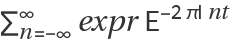.
ResourceFunction["InverseFourierCoefficient"] returns a periodic function of t with default period 1.
Different choices for the definition of the Fourier exponential series representation can be specified using the option FourierParameters.
With the setting FourierParameters{a,b}, the Fourier exponential series representation used by ResourceFunction["InverseFourierCoefficient"] is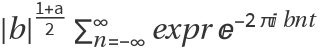, a periodic function of t with period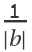.

## Examples

### Basic Examples

Find a function with a given Fourier series:

 In:=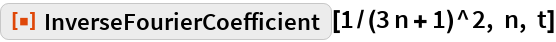Out=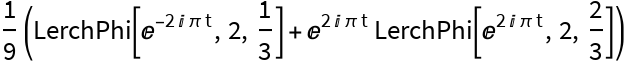Compare with the answer from a numerical approximation:

 In:=Out=In:=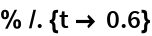Out=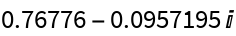In:=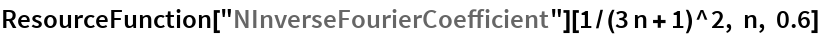Out=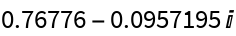## Requirements

Wolfram Language 11.3 (March 2018) or above